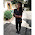## What is a Histogram graph in 7 QC Tools?

→ It is a type of bar chart representing the frequency distribution of the data from a process.
→ Only one parameter can be used for constructing this chart.
→ It is a frequency distribution tool of the Basic 7 QC Tools for the process improvement.
→ The parameter must be variable data, i.e., measured on a continuous scale. ( eg. weight, time, temperature, dimensions, speed, etc.)
→ This tool is used in the DMAIC Methodology of Six Sigma Project

### What is a histogram used for?

• It is used for showing a large data set graphically
• Problem forecasting
• Study the variation in the ongoing process

### Key Concept of Histogram in 7 QC Tools:

➨ The Key Concept is:
⇢ Data always have a variation
⇢ Variation has Pattern
⇢ Patterns can be seen easily when summarized pictorially

## How to Make a Histogram?

→ Seven Steps are mentioned below for making the histograph:
1. Data Collection
2. Compute the Range
3. Determine the numbers of intervals
4. Determine the interval width
5. Summarize the record
6. Construct the Graph
7. Analyze the pattern of the chart

####  Data Collection:

→ For better analysis and forecasting of process collect at least 100 record points.
→ Here we take an example of 150 record points for making a chart.

####  Compute the Range:

→ Largest Value        = 442
→ Smallest Value      = 204
→ Range of Values   =  442-204  = 238

####  Determine the No. of intervals required:

→ This step is for determining the no. of intervals required.

####  Determine the interval width:

→ Step 4 is for determining the width of intervals to construct.

####  Summarize the record:

→ In Step 5 we will summarize the data as per the interval and count the frequency of data as per range value for construction of the_graph.

####  Construct the Graph:

→ Now we will construct the_graph by mentioning the Title,
→ Y-axis/Vertical axis = Interval width, X-axis/Horizontal axis = Interval width,
→ Graph - Trendline and Legend (if applicable).

➨ Five Important Parameters in Histogram:
⇢ Title
⇢ Y-axis/Vertical axis = Interval width
⇢ X-axis/Horizontal axis = Interval width
⇢ Graph - Trendline
⇢ Legend (if applicable)
⇢ For a better understanding of these 5 parameters study below chart:

####  Analyze the Graph pattern:

→ Interpretation of the Graph
⇢ Central Tendency
⇢ Process Variation
⇢ Histogram Shape
⇢ Process Capability (Comparison with the specification)

## Examples of Histogram Graphs

### Types of Histogram Patterns

→ Various types of Histograms based on patterns are mentioned below
➨ [A] Normal Distribution:
⇢ Bell Shaped Curve
⇢ A peak in the middle

➨ [B] Skewed Distribution:
⇢ A peak is off-center either right or left
⇢ Analysis of very pure products is skewed i.e. product cannot be more than 100%.
⇢ The range is not set properly for operation

➨ [C] Double-Peaked / Bi-modal:
⇢ Two Bell Shaped distributions.
⇢ Maybe a combination of records of two processes or two shifts.

➨ [D] Multi Peaked / Multi-modal:
⇢ Maybe readings of several processes are plotted on the same graph.
⇢ Range selection is not proper.

➨ [E] Edge Peaked:
⇢ Borderline doubt for inspection and take it as ok.
⇢ Instrument error.

➨ [F] Truncated or Heart-cut:
⇢ The supplier might mix apart within spec and out of spec. limit.
⇢ 100% of Incoming inspection and screening of mixed parts.

### Benefits of Histogram:

→ Summarize Large data set Graphically
→ Confirms measurements to Specification
→ Excellent problem forecasting tool in the process
→ Assist to decision making in the ongoing process

2. Cause & Effect Diagram (Fishbone or Ishikawa)

👉 For a regular update :

1.Very well explained. @Nikunjbhoraniya.

1.Thank you for your valuable feedback it will motivate us

2.Dear sir pls explain the difference bet"n common causes and special causes with example

1.Thank you for your suggestion we have already explained with example in SPC article so please check our article on SPC

3.Thnqqq soo much.. very helpful and systematic

1.Thank you for your kind words

4.Thanks for sharing. Refreshing those QC tools in a very systematic manner.

5.Very nicely explained thank you very much sir!

1.Thank you very much for your valuable feedback